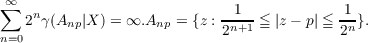#### Vol. 51, No. 2, 1974

 Download this articleFor screen For printingRecent Issues Vol. 325: 1  2 Vol. 324: 1  2 Vol. 323: 1  2 Vol. 322: 1  2 Vol. 321: 1  2 Vol. 320: 1  2 Vol. 319: 1  2 Vol. 318: 1  2Online Archive Volume: Issue:The Journal Subscriptions Editorial Board Officers Contacts Submission Guidelines Submission Form Policies for Authors ISSN: 1945-5844 (e-only) ISSN: 0030-8730 (print) Special Issues Author Index To Appear Other MSP Journals
A counterexample to a conjecture on an integral condition for determining peak points (counterexample concerning peak points)

### Alfred Peter Hallstrom

Vol. 51 (1974), No. 2, 455–457
##### Abstract

Let X be a compact plane set. Denote by R(X) the uniform algebra generated by the rational functions with poles off X and by H(X) the space of functions harmonic in a neighborhood of X endowed with the sup norm. A point p ∂X is a peak point for R(X) if there exists a function f R(X) such that f(p) = 1 and |f(x)| < 1 if xp. Moreover, p is a peak point for H(X) (consider Ref) and hence, by a theorem of Keldysh, p is a regular point for the Dirichlet problem. Conditions which determine whether or not a point is a peak point for R(X) are thus of interest in harmonic analysis. Melnikov has given a necessary and sufficient condition that p be a peak point for R(X) in terms of analytic capacity, γ; namely p is a peak point for R(X) if and only ifAnalytic capacity is generally difficult to compute, so it is desirable to obtain more computable types of conditions. Let Xc = C|X and I = {t [0,1] : z Xc and |z| = t}. In this note the following conjecture, which can be found in Zalcman’s Springer Lecture Notes and which is true for certain sets X, is shown to be false in general:

Conjecture. If It1dt = then 0 is a peak point for R(X).

Primary: 30A98
##### Milestones